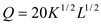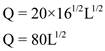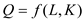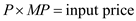## Quiz 8 : Production and Cost in the Short RunLooking for Economics Homework Help?# Quiz 8 : Production and Cost in the Short Run

The following explains about various costs of Exquisite Portraits Inc. (A) The fixed cost, quasi fixed cost and variable cost are as follows: - Fixed costs are the costs that are not dependent on the level of output and are incurred despite output being zero. Here, lease of equipment (\$1000) and rent (\$1400) are fixed cost. The total fixed cost is \$2400. - Quasi fixed costs are incurred at a fixed amount when output is not equal to zero. Here quasi fixed cost is \$45 for electricity and coffee. - Variable costs are the costs that vary with level of output. Here variable cost is electricity for studio that varies according to hours of usage. (B) The sunk costs are the costs that cannot be recovered once incurred. - Here sunk costs are business card cost (\$200), Yellow pages cost (\$1000), license cost (\$250) and equipment lease cost (\$1000 for 4 months). The total sunk cost is \$ 5450. - She can avoid cost of electricity and rent. (C) While making decision sunk cost should not be considered. She should see whether the future revenues are greater the future costs. (D) No, sunk cost does not play any role in decision making process. Though it affects the annual profit of the company, it is not seen as a major determinant of decision making.

The following is the production function of a firm:(A) The short production is when the capital is held constant. Here K=16. The short run production Q function would be:(B) The average product of labor function is derived by dividing the production function by labor input:(C) The marginal product of labor function is derived by differentiating the production function with respect to labor (L)(D) The marginal product diminishes with all increasing level of input. If one increases the denominator i.e. labor, the marginal product falls.

The production function is the relation between inputs such as labor (L) and capital (K) output (Q) :Differentiation the above equation gives marginal product. Marginal product means that marginal change in output for a unit change in input. The marginal product is always falling curve. Diminishing return is the stage of production which the increase in input should be stopped. But even in this stage, the employer can still hire a new person if the value of marginal product is above the wage rate. Diminishing returns occurs when a unit increase in input gives decreased return in output. This happens when the marginal product starts going in negative value. If new employee is added which results in fall in marginal product but rise in total product, then such worker should be hired. The labor should be increased till the marginal product reaches zero. This is highest point of the total product. After this point the marginal product becomes negative and total product starts falling. The rule is that:. If the wage rate declines, it will hire more people. Or the value of MP increase due to an increase in product price, it can still hire new person. That's why the demand for inputs is downward sloping.